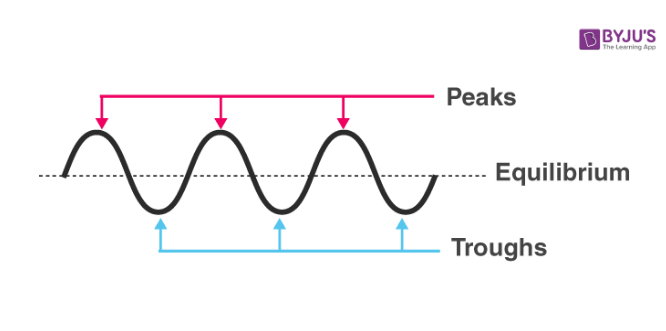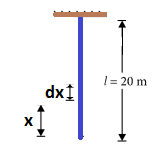# JEE Main Waves on String Previous Year Questions with Solutions

A string can be used to understand the properties of a wave. Imagine, one end of the string is fixed and you are holding the other end of the string. Now, if the string is moved up and down, a wave is formed on it. The type of wave formed is called the transverse wave. A transverse wave is defined as a wave where the movement of the particles of the medium is perpendicular to the direction of the propagation of the wave. In this example, the string acts as the medium.

Transverse waves form peaks and troughs. The top point of the wave is called crest or peak. The bottom point of the wave is called trough.Velocity of the wave on a string Velocity is the distance travelled by the time taken. In waves, this is found by dividing the wavelength by the period: v=λ/T, where 1/T = frequency(f)

## JEE Main Previous Year Solved Questions on Waves on String

Q1: A wave y = a sin(ωt – kx) on a string meets with another wave producing a node at x = 0. Then the equation of the unknown wave is

(a) y = asin(ωt + kx)

(b) y = –asin(ωt + kx)

(c) y = asin(ωt – kx)

(d) y = –asin(ωt – kx)

Solution

Consider option (a)

Stationary wave: Y = asin(ωt + kx) + asin(ωt – kx)

when, x = 0, Y is not zero.

The option is not acceptable.

Consider option (b)

Stationary wave: Y = asin(ωt – kx) – asin(ωt + kx) At x = 0, Y = a sinωt – asinωt = zero

This option holds good.

Option (c) gives Y = 2asin(ωt – kx) At x = 0, Y is not zero

Option (d) gives Y = 0

Hence only option (b) holds good

Answer: (b) y = –asin(ωt + kx)

Q2: The displacement y of a wave travelling in the x-direction is given by

y = 10-4sin(600t – 2x+ π/3) metre,

where x is expressed in metre and t in second. The speed of the wave-motion, in m s–1 is (a) 300

(b) 600

(c) 1200

(d) 200

Solution

Given wave equation: y= 10-4 sin (600t – 2x + π/3)m

Standard wave equation: y = asin(t – kx + Φ)

Compare them

Angular speed = ω = 600 sec-1

Propagation constant = k = 2m-1

ω/k = (2πf)/(2π/λ) = fλ = velocity

Since velocity = ω/k = 600/2 = 300 m/sec

Q3: A string is stretched between fixed points separated by 75 cm. It is observed to have resonant frequencies of 420 Hz and 315 Hz. There are no other resonant frequencies between these two. Then, the lowest resonant frequency for this string is

(a) 10.5 Hz

(b) 105 Hz

(c) 1.05 Hz

(d) 1050 Hz.

Solution

For the string fixed at both the ends, resonant frequency are given by f = nv/2L, where symbols have their usual meanings. lt is given that 315 Hz and 420 Hz are two consecutive resonant frequencies, let these be nth and (n + 1)th harmonics.

315 = nv/2L———(1)

420 = (n+1)v/2L —(2)

Dividing equa (1) by equa (2) we get

n = 3

From equa (1), lowest resonant frequency

f0 = v/2L = 315/3 = 105 Hz

Q4: The transverse displacement y(x,t) of a wave on a string is given by

$y(x,t)=e^{-(ax^{2}+bt^{2}+2\sqrt{ab}xt)}$

This represents a

(a) wave moving in +x-direction with speed $\sqrt{\frac{a}{b}}$

(b) wave moving in –x-direction with speed $\sqrt{\frac{b}{a}}$

(c) standing wave of frequency $\sqrt{b}$

(d) standing wave of frequency 1/ $\sqrt{b}$

Solution

Given the equation $y(x,t)=e^{-(ax^{2}+bt^{2}+2\sqrt{ab}xt)}$——–(1)

$y(x,t)=e^{-(\sqrt{a}x+\sqrt{b}t)}$2

Comparing equation (1) with standard equation y(x, t) = f (ax + bt)

As there is a positive sign between x and t terms, hence wave travel in –x direction.

Wave speed= Coefficient of t/Coefficient of x = $\sqrt{\frac{b}{a}}$

Answer: (b) wave moving in –x-direction with speed $\sqrt{\frac{b}{a}}$

Q5: The equation of a wave on a string of linear mass density 0.04 kg m–1 is given by

$y=0.02(m)sin2\pi \left ( \frac{t}{0.04(s)}-\frac{x}{0.50(m)} \right )$

The tension in the string is

(a) 6.25 N

(b) 4.0 N

(c) 12.5 N

(d) 0.5 N

Solution

Here, linear mass density μ = 0.04 kg m–1

The given equation of a wave is $y = 0.02sin\left [ 2\pi \left ( \frac{t}{0.04}-\frac{x}{0.50} \right ) \right ]$

Compare it with the standard wave equation y = Asin(ωt – kx)

Wave velocity, v = ω/k= (2π/ 0.04)/(2π/ 0.5) ms-1——-(1)

Also, $v = \sqrt{\frac{T}{\mu }}$——-(2)

Here, T is the tension in the string and μ is the linear mass density

Equating equations (1) and (2), we get

$\frac{\omega }{k}= \sqrt{\frac{T}{\mu }}$

T = μω2/k2

T = [ 0.04 x (2π/0.04)2] /(2π/0.5)2= 6.25 N

Q6: A wave travelling along the x-axis is described by the equation y(x, t) = 0.005 cos(αx –β t). If the wavelength and the time period of the wave are 0.08 m and 2.0 s, respectively, then α and β in appropriate units are

(a) α = 12.50π, β = π/2.0

(b) α = 25.00π, β = π

(c) α = 0.08/π, β = 2.0/π

(d) α = 0.04/π, β =1.0/π

Solution

The wave travelling along the x-axis is given by

y(x,t) = 0.005 cos(αx – βt)

Therefore,α = k = 2π/λ As λ = 0.08 m

α = 2π/0.08 = π/0.04 ⇒ α = (π/4 ) x 100 = 25.00π

ω = β ⇒2π/2 =π

∴ α = 25.00π

β = π

Answer: (b) α = 25.00π, β = π

Q7: A uniform string of length 20 m is suspended from a rigid support. A short wave pulse is introduced at its lowest end. It starts moving up the string. The time taken to reach the support is (take g = 10 m s–2)

(a) 5π $\sqrt{5}$s

(b) 2s

(c) 2$\sqrt{2}$s

(d) $\sqrt{5}$

Solution

A uniform string of length 20 m is suspended from a rigid support.As μ is mass per unit length of the rope, then μ =m/L

As $v = \sqrt{\frac{T}{\mu }}$

dx/dt = $\frac{dx}{dt}= \sqrt{\frac{mgx/L}{m/L}} = \sqrt{g}\sqrt{x}$ $\frac{dx}{\sqrt{x}}= \sqrt{g}dt$

Integrating, on both sides, we get

$\int_{0}^{L}x^{-1/2}dx = \sqrt{g}\int_{0}^{L}dt$ $t = 2\sqrt{L}/\sqrt{g} = 2 \times \sqrt{\frac{20}{10}}=2\sqrt{2}s$

Answer: (c) 2$\sqrt{2}$s

Q8: A sonometer wire of length 1.5 m is made of steel. The tension in it produces an elastic strain of 1%. What is the fundamental frequency of steel if the density and elasticity of steel are 7.7 × 103 kg/m3 and 2.2 × 1011 N/m2 respectively?

(a) 770 Hz

(b) 188.5 Hz

(c) 178.2 Hz

(d) 200.5

Solution

Frequency ,f = v/2l

$\frac{1}{2l}\sqrt{\frac{T}{\mu }}=\frac{1}{2l}\sqrt{\frac{T}{Ad}}$

Where, T is tension in the wire and μ is the mass per unit length of wire.

Also, Young’s modulus, Y = Tl/AΔl

T/A = YΔl/l

Substituting this value in frequency expression, we get

$f = \frac{1}{2l}\sqrt{\frac{y\Delta l}{ld}}$

Given, l = 1.5 m, Δl/l = 0.01

d= 7.7 x 103 kg/m3

Y= 2.2 x 1011 N/m2

Substituting these values we have

$f = \frac{1}{2l}\sqrt{\frac{2.2 \times 10^{11}\times 0.01}{7.7\times 10^{3}}}$

f = 178.2 Hz

Q9: Two sitar strings, A and B, playing the note ‘Dha’ are slightly out of tune and produce beats of frequency 5 Hz. The tension of the string B is slightly increased and the beat frequency is found to decrease by 3 Hz. If the frequency of A is 425 Hz, the original frequency of B is

(a) 428 Hz

(b) 430 Hz

(c) 420 Hz

(d) 422 Hz

Solution

Frequency of sitar string B is either 420 Hz or 430 Hz. As tension in string B is increased, its frequency will increase. If the frequency is 430 Hz, the beat frequency will increase. If the frequency is 420 Hz, beat frequency will decrease, hence the correct answer is 420 Hz.

Q10: A standing wave is formed by the superposition of two waves travelling in opposite directions. The transverse displacement is given by

y(x,t)= 0.5sin[(5π/4)x]cos(200πt)

What is the speed of the travelling wave moving in the positive x-direction?

(x and t are in meter and second, respectively)

(a) 180 m/s

(b) 160 m/s

(c) 120 m/s

(d) 90 m/s

Solution

Given y(x,t)= 0.5sin[(5π/4)x]cos(200πt)

Comparing this equation with standard equation of standing wave, y(x, t) = 2a sin kx cosωt, we get, k = 5π/4 rad/m, ω = 200 rad/s

Speed of the travelling wave, v = ω/k = 200π/(5π/4) = 160 m/s

Q11: Length of a string tied to two rigid supports is 40 cm. Maximum length (wavelength in cm) of a stationary wave produced on it is

(a) 20

(b) 80

(c) 40

(d) 120

Solution

λmax/2 = 40 ⇒ λmax = 80 cm

Q12: Statement-1:Two longitudinal waves given by equations y1(x,t)=2asin(ωt – kx) and y2(x,t)=2asin(2ωt – 2kx) will have equal intensity.

Statement-2: Intensity of waves of given frequency in the same medium is proportional to square of amplitude only.

(a) Statement-1 is false, statement-2 is true

(b) Statement-1 is true, statement-2 is false

(c) Statement-1 is true, statement-2 true; statement-2 is the correct explanation of statement-1

(d) Statement-1 is true, statement-2 is true; statement -2 is not correct explanation of statement-1

Solution

y1(x,t) = 2asin(ωt – kx)

y2(x,t) = 2asin(2ωt – 2kx)

But Intensity, I = ½(ρω2A2v)

Here, ρ = density of the medium,

A= amplitude

v = velocity of the wave

Intensity depends upon amplitude, frequency and velocity of the wave.

Also, I1 = I2

Answer: (d) Statement-1 is true, statement-2 is true; statement -2 is not correct explanation of statement-1

Q13: A pipe open at both ends has a fundamental frequency ‘f’ in air. The pipe is dipped vertically in water so that half of it is in water. The fundamental frequency of the air column is now

(a) f

(b) f/2

(c) 3f/4

(d) 2f

Solution

When the pipe is open at both ends

λ/2 = l

λ = 2l

v = fλ

f = v/λ = v/2l ———-(1)

When the pipe is dipped vertically in the water, so that half of the pipe is in water

λ/4 = l/2

λ = 2l ⇒v = f’λ

f’ = v/λ = v/2l =f——-(2)

Thus, the fundamental frequency of the air column is now,

f = f’

Q14: A string of length 1 m and mass 5g is fixed at both ends. The tension in the string is 8.0 N. The string is set into vibration using an external vibrator of frequency 100 Hz. The separation between successive nodes on the string is close to

1. 10 cm
2. 33.3 cm
3. 16.6 cm
4. 20.0 cm

Solution

Velocity of the wave on the string

V = $\sqrt{\frac{t}{\mu }}$ = $\sqrt{\frac{8}{5}\times 100}$

Here, T = tension and μ = mass/length

Wavelength of the wave λ = v/n = 40/100

Separation between successive nodes,

λ/2 = 40/(2x 100) = 20 cm

Q15: Equation of a travelling wave on a stretched string of linear density 5g/m is y=0.03sin(450t – 9x) where distance and time are measured in SI units. The tension in the string is

(a) 10 N

(b) 7.5 N

(c) 12.5 N

(d) 5 N

Solution

We have given,

y = 0.03sin(450t – 9x)

Comparing it with standard equation of wave, we get

ω = 450k=9

v = ω/k = 450/9 = 50m/s

Velocity of the travelling wave on a string is given by

v = $\sqrt{\frac{t}{\mu }}$ = T/μ = 2500

μ = linear mass density

T = 2500 x 5 x 10-3

T = 12.5 N